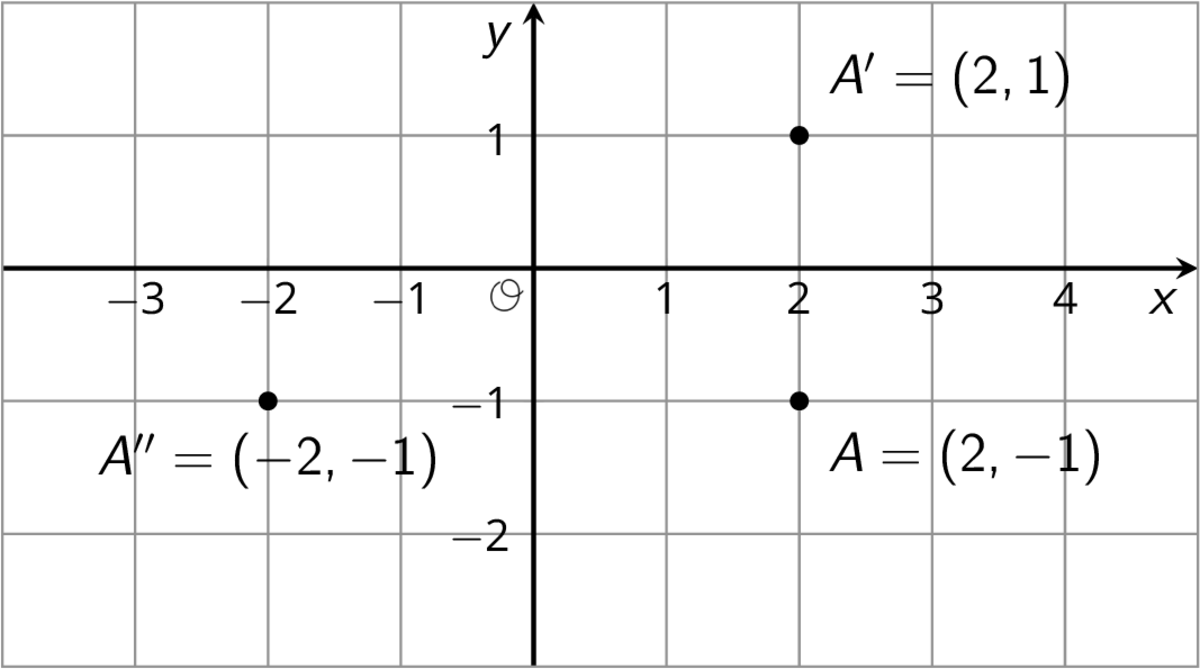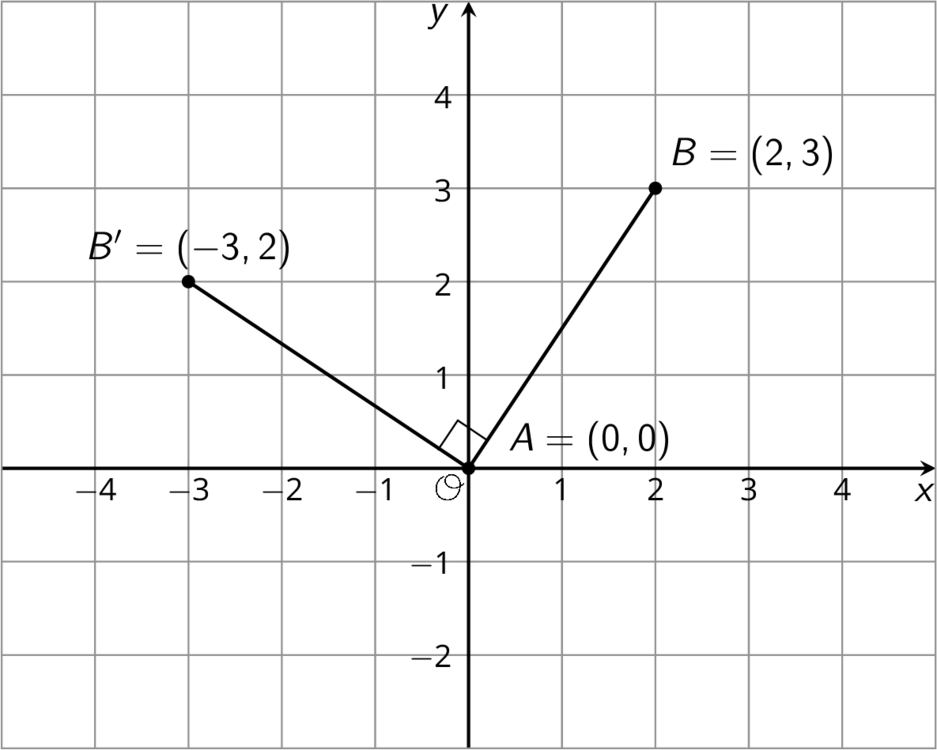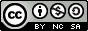Reflections, Translations and Grids

Rigid HW

1.5 Coordinate MovesUNIT 1 • LESSON 5 COORDINATE MOVES

WHAT YOU WILL LEARNIn this lesson, I will transform some figures and see what happens to the coordinates of points.I can...
• Apply transformations to a line segment on a coordinate grid by applying the transformation to each endpoint and then connecting the dots.
• Apply transformations on a coordinate grid.
I will know I learned by...
• Demonstrating that I can apply transformations to points on a grid if I know their coordinates.
FAMILY MATERIALS:To review or build a deeper understanding of the math concepts, skills, and practices in this lesson, visit the Family Materials provided by Illustrative Mathematics Open-Up Resources. (Links to an external site.)Links to an external site.Select all of the translations that take Triangle T to Triangle U. There may be more than one correct answer.1. Translate (-3,0)(-3,0) to (1,2)(1,2).
2. Translate (2,1)(2,1) to (-2,-1)(-2,-1).
3. Translate (-4,-3)(-4,-3) to (0,-1)(0,-1).
4. Translate (1,2)(1,2) to (2,1)(2,1).
1. Five points are plotted on the coordinate plane.Geogebra Applet (Links to an external site.)Links to an external site.   (Links to an external site.)Links to an external site.
• Using the Pen tool or the Text tool, label each with its coordinates.
• Using the xx-axis as the line of reflection, plot the image of each point.
• Label the image of each point using a letter. For example, the image of point AA should be labeled A′A′.
• Label each with its coordinates.
2. If the point (13,10)(13,10) were reflected using the xx-axis as the line of reflection, what would be coordinates of the image? What about (13,-20)(13,-20)? (13,570)(13,570)? Explain how you know.
3. The point RR has coordinates (3,2)(3,2).
• Without graphing, predict the coordinates of the image of point RR if point RR were reflected using the yy-axis as the line of reflection.
• Check your answer by finding the image of RR on the graph.• Label the image of point RR as R′R′.
• What are the coordinates of R′R′?
4. Suppose you reflect a point using the yy-axis as line of reflection. How would you describe its image?
The applet has instructions for the first 3 questions built into it. Move the slider marked “question” when you are ready to answer the next one. Pause before using the applet to show the transformation described in each question to predict where the new coordinates will be.Apply each of the following transformations to segment ABAB. Use the Pen tool to record the coordinates.
1. Rotate segment ABAB 90 degrees counterclockwise around center BB by moving the slider marked 0 degrees. The image of AA is named CC. What are the coordinates of CC?
2. Rotate segment ABAB 90 degrees counterclockwise around center AA by moving the slider marked 0 degrees. The image of BB is named DD. What are the coordinates of DD?
3. Rotate segment ABAB 90 degrees clockwise around (0,0)(0,0) by moving the slider marked 0 degrees. The image of AA is named EE and the image of BBis named FF. What are the coordinates of BB and FF?
4. Compare the two 90-degree counterclockwise rotations of segment ABAB. What is the same about the images of these rotations? What is different?
Geogebra Applet (Links to an external site.)Links to an external site.Suppose EFEF and GHGH are line segments of the same length.  Describe a sequence of transformations that moves EFEF to GHGH.We can use coordinates to describe points and find patterns in the coordinates of transformed points.We can describe a translation by expressing it as a sequence of horizontal and vertical translations.  For example, segment AB is translated right 3 and down 2.Reflecting a point across an axis changes the sign of one coordinate. For example, reflecting the point A whose coordinates are (2,-1) across the x-axis changes the sign of the y-coordinate, making its image the point A′ whose coordinates are (2,1). Reflecting the point A across the y-axis changes the sign of the x-coordinate, making the image the point A″ whose coordinates are (-2,-1).Reflections across other lines are more complex to describe.We don’t have the tools yet to describe rotations in terms of coordinates in general. Here is an example of a 90∘ rotation with center (0,0) in a counterclockwise direction.Point A has coordinates (0,0). Segment AB was rotated 90∘ counterclockwise around A. Point B with coordinates (2,3) rotates to point B′ whose coordinates are (-3,2).All Illustrative Mathematics Open Up Resources can be Downloaded for free at openupresources.org (Links to an external site.)Links to an external site.. Any additional HCPSS content is offered under a CC Attribution Non-Commercial Share AlikeLinks to an external site. license.